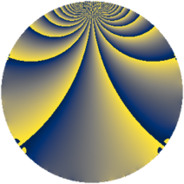Properties

 Label 637.2.rLevel $637$ Weight $2$ Character orbit 637.r Rep. character $\chi_{637}(116,\cdot)$ Character field $\Q(\zeta_{6})$ Dimension $84$ Newform subspaces $7$ Sturm bound $130$ Trace bound $12$

Related objects

Defining parameters

 Level: $$N$$ $$=$$ $$637 = 7^{2} \cdot 13$$ Weight: $$k$$ $$=$$ $$2$$ Character orbit: $$[\chi]$$ $$=$$ 637.r (of order $$6$$ and degree $$2$$) Character conductor: $$\operatorname{cond}(\chi)$$ $$=$$ $$91$$ Character field: $$\Q(\zeta_{6})$$ Newform subspaces: $$7$$ Sturm bound: $$130$$ Trace bound: $$12$$ Distinguishing $$T_p$$: $$2$$, $$3$$

Dimensions

The following table gives the dimensions of various subspaces of $$M_{2}(637, [\chi])$$.

Total New Old
Modular forms 148 100 48
Cusp forms 116 84 32
Eisenstein series 32 16 16

Trace form

 $$84 q + 4 q^{3} + 38 q^{4} - 22 q^{9} + O(q^{10})$$ $$84 q + 4 q^{3} + 38 q^{4} - 22 q^{9} + 6 q^{10} - 18 q^{12} + 12 q^{13} - 34 q^{16} - 8 q^{17} + 12 q^{22} + 22 q^{23} + 28 q^{25} + 6 q^{26} - 32 q^{27} + 4 q^{29} - 10 q^{30} + 24 q^{36} - 34 q^{38} - 14 q^{39} + 4 q^{40} - 116 q^{43} - 36 q^{48} + 24 q^{51} + 42 q^{52} - 22 q^{53} - 24 q^{55} + 12 q^{61} - 44 q^{62} - 176 q^{64} + 20 q^{65} - 2 q^{66} + 2 q^{68} + 56 q^{69} - 54 q^{74} - 8 q^{75} + 36 q^{78} - 62 q^{79} + 38 q^{81} + 16 q^{82} + 68 q^{87} - 52 q^{88} + 216 q^{90} - 28 q^{92} + 26 q^{94} - 34 q^{95} + O(q^{100})$$

Decomposition of $$S_{2}^{\mathrm{new}}(637, [\chi])$$ into newform subspaces

Label Dim. $$A$$ Field CM Traces $q$-expansion
$a_{2}$ $a_{3}$ $a_{5}$ $a_{7}$
637.2.r.a $4$ $5.086$ $$\Q(\zeta_{12})$$ None $$0$$ $$-4$$ $$0$$ $$0$$ $$q+2\zeta_{12}q^{2}-2\zeta_{12}^{2}q^{3}+2\zeta_{12}^{2}q^{4}+\cdots$$
637.2.r.b $4$ $5.086$ $$\Q(\sqrt{-3}, \sqrt{-13})$$ $$\Q(\sqrt{-91})$$ $$0$$ $$0$$ $$0$$ $$0$$ $$q-2\beta _{2}q^{4}-\beta _{1}q^{5}+(3-3\beta _{2})q^{9}+\beta _{3}q^{13}+\cdots$$
637.2.r.c $4$ $5.086$ $$\Q(\zeta_{12})$$ None $$0$$ $$4$$ $$0$$ $$0$$ $$q+2\zeta_{12}q^{2}+2\zeta_{12}^{2}q^{3}+2\zeta_{12}^{2}q^{4}+\cdots$$
637.2.r.d $12$ $5.086$ 12.0.$$\cdots$$.1 None $$0$$ $$0$$ $$0$$ $$0$$ $$q+(\beta _{3}+\beta _{4}+\beta _{6}+\beta _{7})q^{2}+\beta _{11}q^{3}+\cdots$$
637.2.r.e $12$ $5.086$ 12.0.$$\cdots$$.1 None $$0$$ $$0$$ $$0$$ $$0$$ $$q+(\beta _{3}+\beta _{4}+\beta _{6}+\beta _{7})q^{2}-\beta _{11}q^{3}+\cdots$$
637.2.r.f $16$ $5.086$ $$\mathbb{Q}[x]/(x^{16} - \cdots)$$ None $$0$$ $$4$$ $$0$$ $$0$$ $$q+(-\beta _{1}-\beta _{8})q^{2}+(1-\beta _{4}-\beta _{7})q^{3}+\cdots$$
637.2.r.g $32$ $5.086$ None $$0$$ $$0$$ $$0$$ $$0$$

Decomposition of $$S_{2}^{\mathrm{old}}(637, [\chi])$$ into lower level spaces

$$S_{2}^{\mathrm{old}}(637, [\chi]) \cong$$ $$S_{2}^{\mathrm{new}}(91, [\chi])$$$$^{\oplus 2}$$